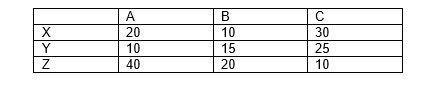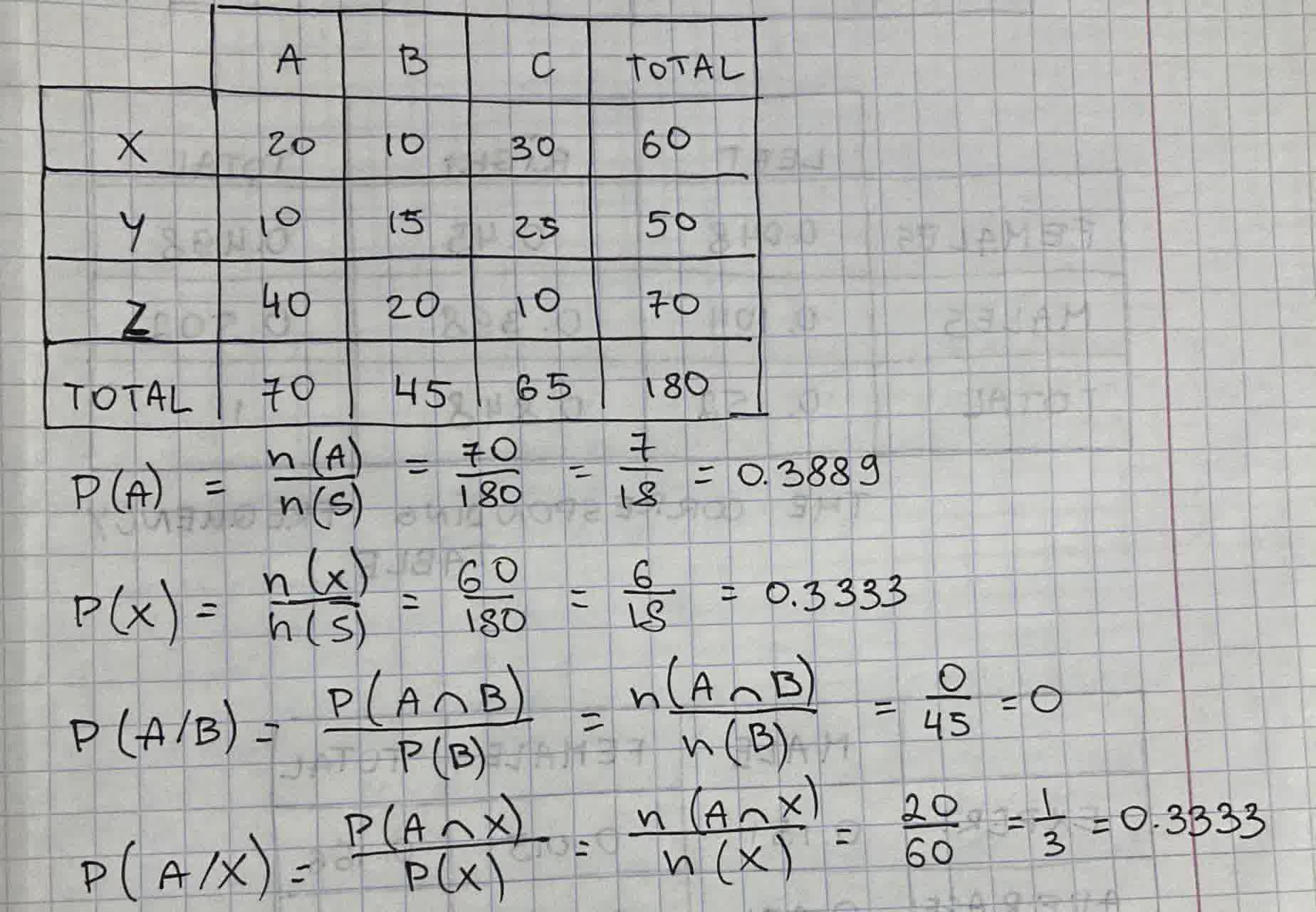# Answer the questions using the table below: P(A) = ? P (A/B) = ? P (X) = ?Caelan 2021-09-08 Answered

Answer the questions using the table below:
$P\left(A\right)=?$
$P\left(\frac{A}{B}\right)=?$
$P\left(X\right)=?$
$P\left(\frac{A}{X}\right)=?$You can still ask an expert for help

## Want to know more about Two-way tables?

• Questions are typically answered in as fast as 30 minutes

Solve your problem for the price of one coffee

• Math expert for every subject
• Pay only if we can solve itTuthornt

Find total: